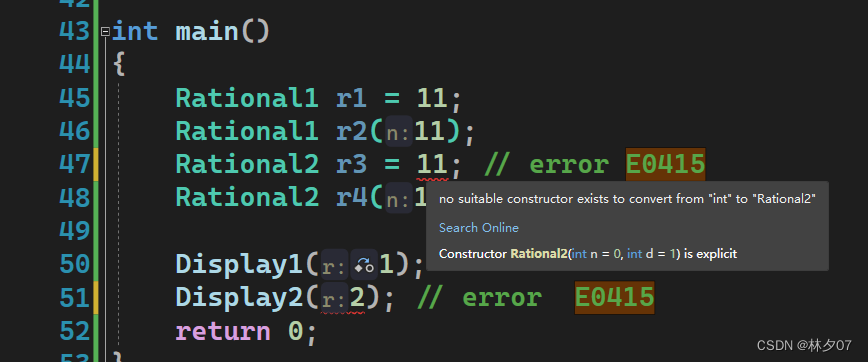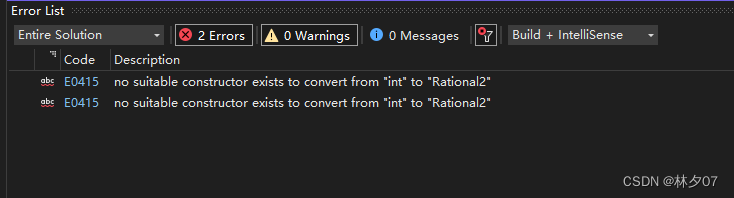# [Solved] Error:E0415 no suitable constructor exists to convert from “int“ to “Rational“

Scene:

The problem is that the constructor for is missing or is declared as explicit.

Please refer to the following scenario.

``````#include <iostream>

using std::cout;
using std::endl;

class Rational1
{
public:
Rational1(int n = 0, int d = 1):num(n), den(d)
{
cout << __func__ << "(" << num << "/" << den << ")" << endl;
}

public:
int num;
int den;
};

class Rational2
{
public:
explicit Rational2(int n = 0, int d = 1) :num(n), den(d)
{
cout << __func__ << "(" << num << "/" << den << ")" << endl;
}

public:
int num;
int den;
};

void Display1(Rational1 r)
{
cout << __func__ << endl;
}

void Display2(Rational2 r)
{
cout << __func__ << endl;
}

int main()
{
Rational1 r1 = 11;
Rational1 r2(11);
Rational2 r3 = 11; // error E0415
Rational2 r4(11);

Display1(1);
Display2(2); // error  E0415
return 0;
}
``````

Explicit keyword

1. Specifies that the constructor or conversion function (from C++11) is explicit, that is, it cannot be used for implicit conversion and copy initialization
2. Explicit can be used with constant expressions The function is explicit if and only if the constant expression evaluates to true (From C++20)

Problem description

Error:E0415 no suitable constructor exists to convert from “int“ to “Rational“Solution:

1. Implement the corresponding constructor yourself. (Recommended)
2. Delete the constructor modified by the explicit keyword. (Not recommended)